# Bending ofBeams - Easy Approach

## Link to: Bending of Beams - Full Theory

Getting AcquaintedCalculating the bending of beams was a time-honored centerpiece in the early teaching of "technical mechanics", something every budding engineer and scientist had to go through as a kind of initiation rite. Many didn't make it. Mastering beam-bending is just not something done on the back of an envelope, while having a beer or two. You really need to apply yourself to pass those exams.
Let's first look at the enormity of the task and then cut it down to pieces we can handle. The figure below shows what it is all about under rather simple circumstances. We have what the illuminati call a "beam", solidly fixed at one end. It just as well could be a sword seen edge-on. Some forces act on our beam; as a result it bends. Let's look at the major points connected to this one by one: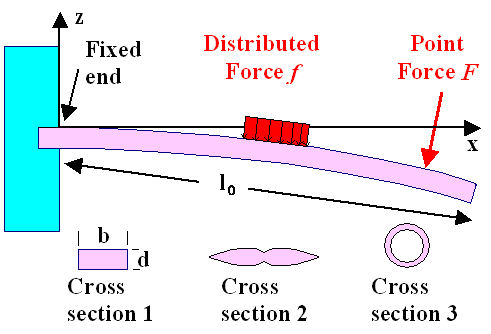Basic bending beam experiment. The cross-section can have any shape but is the same everywhere.First, let's define a bit more precisely what I mean with the word "beam". "Beam" shall be the generic name for any longish object with some cross-section that is the same everywhere along its length l. Moreover, the length l must be considerably larger than the dimensions of the cross-section.
Since the cross-section of most swords changes with the length, those swords are not proper beams but at best approximate beams.Second, let's define "bending". If you bend something, in particular a beam, you deform it mechanically. As far as the word bending is concerned here, we restrict ourselves to purely elastic deformations anywhere in the beam. Take off the forces acting on the beam above, and it must be perfectly straight again.Third, we look at the forces that can bend a beam. We allow all kinds of forces acting anywhere on the beam - as longs as they act in the z-direction only. Otherwise we have two bending beam problems - bending in the z-direction and in the y-direction perpendicular to the drawing plane. Applying forces in the x-direction would mean a tensile (or compressive) test, so we don't allow them either. Of course, the forces acting on real beams or swords do not care about those restrictions but I'm looking at simple beam bending here.
Forces could be point forces, like the force your sword experiences when it is hit with the blade of another sword, or distributed forces, as you get them when you put, for example, a weight on your beam as shown above.
Our beam is supposed to bend but not to move otherwise. It will neither rotate nor is it going anywhere, and that means that the sum of all forces and moments must be zero by definition. That is obviously not the case in the picture above. Well, we forgot:Fourth, the boundary conditions. In the picture above, the beam is fixed solidly in some wall. Mathematically that means the deflection z(x), the amount of bending at the coordinate x or simply the bending line, is zero for x=0.
Expressed mathematically, we have as boundary condition: z(x=0)=0, and, for obvious reasons, dz/dx(x=0)=0.
For the mathematically challenged: The derivative dz/dx gives the slope of the bending curve z(x), which must be zero (i.e. it is parallel to the x-axis) next to the wall
These boundary conditions can only be met if forces and moments act on the beam at x=0 that exactly compensate the forces and moments of the external forces. That is quite nice because it means we don't have to worry about that. Simply speaking, if you immobilize one point of your sword somehow (by putting it into a vice) it can't move or rotate by definition, whatever forces act on it somewhere else.Fifth, and not to forget, the beam can have some arbitrary cross-section, including hollow ones.
The three examples shown are already rather special since they are highly symmetric. This is not required, nevertheless we will only consider cross-sections with some symmetry from now on, in order not to boggle the mind too much.Now let's consider the task at hand. We want to calculate z(x), the curve that describes the shape of the bent beam. We allow any length l and cross-sectional shapes of the beam, and all kinds of forces, force distributions, and all kinds of (sensible) boundary conditions.
Even if you are mathematically so challenged that you had to go into banking, you will appreciate that this cannot be an easy task. How do you express the cross-section No 2 above (a sword with a fuller) in equations? What would the boundary conditions be if you try hold the sword in a fixed position with your hands instead of putting it into a vice? If it is hit by another sword you cannot possibly keep the position of hand and sword completely unchanged - in contrast to a (very strong) vice.Well, the task is certainly not easy - but also not quite as difficult as it may appear. Let's do in in two steps and modules. First, in this module, I give a rather simple description of a special situation, which I then will use to derive a few general rules about bending beams. In a second module I will give a more general treatment.

Guessing a Special SolutionThe good news are that I'm not a mathematician. If I would be one, I couldn't have drawn the picture above because it already contains a kind of solution to the problem at hand since it shows (by guessing) how the beam bends. Mathematicians only solve problems without any prior idea of the result and that means without cute little pictures. That's why they often don't actually solve real problems but only discuss in great detail if the problem might have a solution, many solutions - or possibly none.All of us non-mathematicians, however, just know that a beam bends pretty much as shown above for the given situation. We know even more. We know that if we look at a small part of the beam, we can, at least to a good approximation, assume that the bending can locally be described as being circular.
The upper and lower surface of the beam then can be drawn by a compass using two radii that differ by the thickness d. Compared to the length of the undeformed beam, the upper part is now a bit longer, the lower part a bit shorter. We have looked at this before and there can be no doubt that we always have a neutral axis inside the beam, the length of which has not changed. In our simple example we actually have a neutral surface but for more complex cross-sections sticking to a neutral axis (sometimes also called neutral line or centroid) is better since it is more general. This neutral axis (and here the whole neutral surface), also no doubt, will be in the middle of the rectangular beam as drawn below.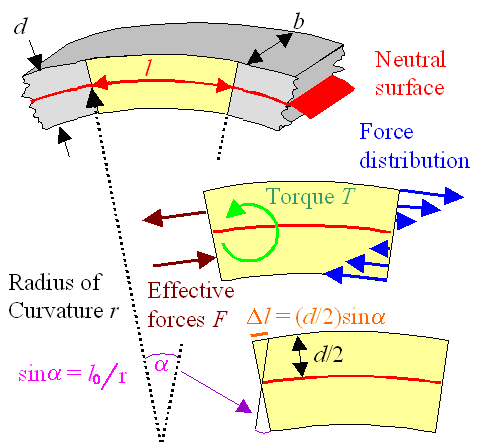A bend segment approximated by circular symmetry Indicated are radius of curvature, force distribution, average forces and resulting torque, and induced length change.Now, in a thought process, we "cut out" the yellow part of the bend beam. To keep it in the shape it had when it still was an integral part of the beam, we must attach forces. The obvious thing to note is that only a distribution of forces as drawn in blue on the right hand side, and the same thing mirrored at the other end (not shown) can produce a deformation as shown. We can always replace the distribution of the blue forces by two point forces F by some smart averaging, and the result will be what is shown in brown on the left-hand end of our beam part.
Now we see that the total effect of the forces is to induce a torsional moment or a torque T with an guessed magnitude of TFd since the distance between the force pair at a guess is d/2, or half the beam thickness as drawn. If you actually calculate the whole thing, the distance would be 2d/3.So far I'm just describing the figure, generating first equations as I go along.
If you keep looking at the figure, you will realize that there is more to see that can be described in simple equations. In particular, the maximum strain right at the top is etmax=d/2r; it is tensile. The maximum strain right at the bottom is ebmax=–d/2r; it is compressive.Why? The picture shows it all. The length of the utmost part is l0 + Dl=l0 + ½d · sina=l0 + (d · l0)/2r). From that we get emax=[l0 + (d · l0/2r) – l0]/l0=d/2r. Voilá!
Equally of course, the strain decreases linearly from the maximum value on the outside to zero at the neutral plane as one moves inside the beam.On average, we guess, the upper or lower part thus experiences an average strain of eavd/4r.As long as we only consider elastic deformations, strain e and stress s are related: s=Y · e; with Y=Young's modulus.The average stress going with our average strain is the average force F divided by half of the cross sectional area bd. In other words: s=(F) / (½bd)=2F / bd=Y · eav=Y · d / 4r.
That gives us for the force F=(Y · d / 4r) · bd / 2=b · d2 ·Y / 8rIt is now easy to realize that in the end it is the torque T=F · d/2 that causes the bending. So let's relate the torque T to the radius of curvature r. With the equation above we get as the final result:

T(r)  =  F · d
2
=  Y · b· d2
8r
·   d
2
=  Y · b · d3
16r

Deducing a Few EssentialsWe have a nice and simple equation. We did a lot of guessing so we cannot expect that this equation is absolutely correct. However, we didn't do anything outrageously wrong so it shouldn't be completely wrong either but a halfway decent approximation. But what does the equation mean?
First, I'm going to tell you what it definitely means, despite the guessing. Then I'm going to discuss the shortcomings of this simple approach and how we can remedy this..The essential deductions we can make are:
• We can always define a radius of curvature r to any segment of a bend beam. The smaller r, the more severe the bending.
• The local radius of curvature is inversely proportional to the local torque or moment. From the equation above we have r(x)=[bd3 / 16] · [Y / T(x)].
• This implies that if you double the width b of your beam, you need to double the torque for the same bending and that is as you would have guessed. If you double the thickness d, you need to increase the torque eight-fold for the same bending. That the thickness counts more than the width is clear, but you could not have guessed the precise relation: it goes with the third power of d!
• The local or induced torque in a bend segment of a beam must have been caused by an external torque of opposite sign, so the total torque is zero. That allows us to deduce qualitatively the deflection curve for a point force as shown below.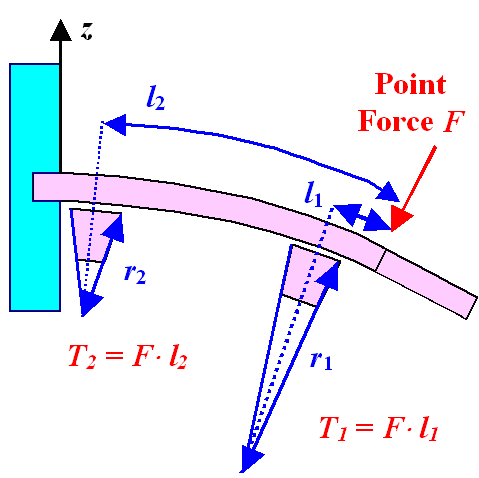Beam bending and local radius of curvatureThe figure illustrates a number of things. First of all, it makes clear that for the point force shown, the local bending is strongest around the clamping of the beam because there you have the highest torque and thus smallest radius of curvature. The radius of curvature thus changes smoothly along the length of the beam up to the location of the force. To the right of the force there is no bending at all in this case (meaning infinite radius of curvature). Note that we have not yet calculated the deflection curve but just seen how it results from local curvatures.
Without illustrating it directly, we can also infer a few other points:If we wouldn't have had a point force but some distributed force, we still can calculate the torque at any point by summing up or integrating over the force distribution. How that is done is not important at this point, all we need to know is that it can be done.
If we would apply a second point force somewhere to the right of the one shown above, we simply sum up the moments of the forces.Isn't it amazing, how much one can deduce about a complex problem without actually solving it "from first principles"? Before I proceed to go beyond guessing, let's look at the problems, oversights and shortcomings of my "guessing" approach.
The first point to make is that little cubes inside our bend beam experience only uniaxial normal stresses. Any small cube inside the beam is just getting a little longer in x-direction; this is shown here in a slightly different context. The strain increases linearly with the distance to the neutral surface.
This is an approximation. Real beams would experience lateral contraction in the upper half and a corresponding widening in the lower. The cross-sectional shape would change from rectangular to trapezoidal. Moreover, the external forces also induce some shear components in the stress, which we neglected. It is clear that looking into that ain't going to be easy, so let me give you the good news right away:
We don't need to do this. While treating beam bending with only uniaxial normal stresses is certainly not quite correct, it is good enough for pretty much all practical cases.
The second point is more serious and will open a large can of worms. In our guessing approach we have assumed a rectangular cross-section, defined by the thickness d times the width or "broadness" b. We have guessed that the neutral plane is right in the middle. If we re-write the final equation for the radius of curvature as above, we can express it as r=(bd3 / 16) · (Y/T) and the factor (bd3 / 16) contains all the information about the cross-section geometry, the assumption about the neutral plane (and thus the direction of the bending), plus the averaging procedures. In other words, it contains all the possible errors and shortcomings of the guessing approach.
It should thus not be a big surprise that the correct value of that factor resulting from proper calculations is actually: (bd3 / 12).
Well, that's just too bad. Nevertheless, the guessing approach wasn't all that bad and certainly worth doing for the simple rectangular cross-section.
The real problem is that for arbitrary cross-sections, the guessing approach doesn't work at all anymore for deducing the value of the factor in front of the Y / T that takes care of the beam geometry. While one could still make a good guess about the position of the neutral surface for the cross-sections given above, you would have a hard time for, e.g., triangular cross-sections and many others.
Worse, you couldn't guess the averages easily either for obvious reasons. So you see the problem:

 How can one account for arbitrary cross-sections in beam bending?Well, our guessing result gives a clear hint: We can keep the general relation r µ (Y / T). All that changes is that the proportionality constant (bd3/16) in our guess work) must be calculated from the real cross-section of the beam. That is actually not very difficult to do (provided you know a bit about integrating in two dimensions).
The result is simply a number that is called the area moment of inertia IA. If there ever was a misnomer, this is it. The area moment of inertia has nothing whatsoever to do with what is called "moment of inertia" for good reasons when you calculate how bodies rotate around some axis. The only connection between the two is that if you write down the integrals for calculating those moments, the mathematical expressions are similar.So all we need to do is to calculate the area moment of inertia I for the cross-section used and to re-write our basic equation as

r  =  IA · Y
T
or       T  =  IA · Y
r

That requires to calculate the position of the neutral axis or centroidfirst. It then will define the coordinate system since we center it on the neutral axis.
Look at the rectangular cross-section in the picture below to get this: The red circle denotes the position of the neutral axis (it runs perpendicular to the picture plane in the x-directions, of course). Since bending about the z-axis (by applying a force in y-direction) will produce results different form bending about the y-axis (by applying a force in z-direction), we need to calculate twoarea moments of inertia, Iy and Iy, to take account of that.
How the centroid and area moments IA are defined, and how they are calculated, is the subject of another module. All we need to know here is that if somebody has calculated it in the past, we don't have to do it ourselves. We just can use the numbers generated.
Here are a few examples; the centroid position is drawn as a red dot.

Iy=Iz=a4/12 Iy=bd3/12 Iy=Iz=pr4/4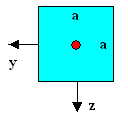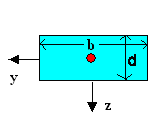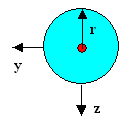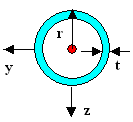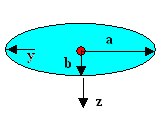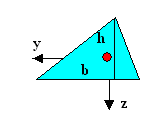Area moments and centroid position for some cross-section. The neutral surface passes through the centroid in the y- or z-direction for bending in the z or y direction, respectively.The red dot denotes the neutral line. For the same Young's modulus Y, the area moments now give the resistance to bending in y- or z- direction (Iz or Iy, respectively. The inde denotes the axis around which the bending occurs). What you can appreciate right away is that a light-weight tube with a somewhat larger radius than a heavy solid circular rod can have the same area moment of inertia and thus resistance to bending. That's why you always have hollow beams (or the
and double
shaped ones) in any structural construction.
Sword cross-sections tend to be more complicated than the ones given above, but with the elliptical one we could at least make an educated guess for swords. That allows us to estimate the strain in the outermost layers as a function of bending and that gives us a last important insight:
As soon as that strain e (or the stress related by s/e=Y) reaches the limit for either plastic deformation or fracture, it is all over. Since we must assume that somewhere along the blade there might be a "weak" spot, it is all over somewhat earlier than what we would calculate.
Finally, I just tell you, that what we learned here can also be carried over to the "buckling of bemas" problem, or the effect that your sword might bend when you try to pierce straight into something. That will be dealt with in a special module

## Link to: Bending of Beams - Full TheoryGalileo GalileiBending of Beams - Full TheoryScience of Uniaxial DeformationArea Moment of Inertia12.2. Static Sword Properties; 12.2.1 Sword BendingBending a Sword into a CircleScience of Deformation12.2.2 Sword Bending: Deformation and Fracture12.3.7 Percussion Point12.3 Dynamic Properties; 12.3.1 Moving a StickBuckling

© H. Föll (Iron, Steel and Swords script)